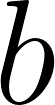×
Log in to StudySoup
Get Full Access to Precalculus Enhanced With Graphing Utilities - 6 Edition - Chapter 1.1 - Problem 5
Join StudySoup for FREE
Get Full Access to Precalculus Enhanced With Graphing Utilities - 6 Edition - Chapter 1.1 - Problem 5

Already have an account? Login here
×
Reset your password

# The area of a triangle whose base is b and whose altitudeISBN: 9780132854351 232

## Solution for problem 5 Chapter 1.1

Precalculus Enhanced with Graphing Utilities | 6th Edition

• Textbook Solutions
• 2901 Step-by-step solutions solved by professors and subject experts
• Get 24/7 help from StudySoup virtual teaching assistantsPrecalculus Enhanced with Graphing Utilities | 6th Edition

4 5 1 380 Reviews
11
4
Problem 5

The area of a triangle whose base is b and whose altitude is h is A = _________. (p. A15)

Step-by-Step Solution:

Problem 5

The area of a triangle whose base is b and whose altitude is h is A = _________. (p. A15)

Step by step solution

Step 1 of 2

Consider the triangle base.

AltitudeStep 2 of 2

##### ISBN: 9780132854351

The answer to “The area of a triangle whose base is b and whose altitude is h is A = _________. (p. A15)” is broken down into a number of easy to follow steps, and 20 words. This textbook survival guide was created for the textbook: Precalculus Enhanced with Graphing Utilities, edition: 6. Since the solution to 5 from 1.1 chapter was answered, more than 301 students have viewed the full step-by-step answer. The full step-by-step solution to problem: 5 from chapter: 1.1 was answered by , our top Math solution expert on 01/11/18, 01:38PM. Precalculus Enhanced with Graphing Utilities was written by and is associated to the ISBN: 9780132854351. This full solution covers the following key subjects: . This expansive textbook survival guide covers 114 chapters, and 8075 solutions.

Unlock Textbook Solution

Enter your email below to unlock your verified solution to:

The area of a triangle whose base is b and whose altitude